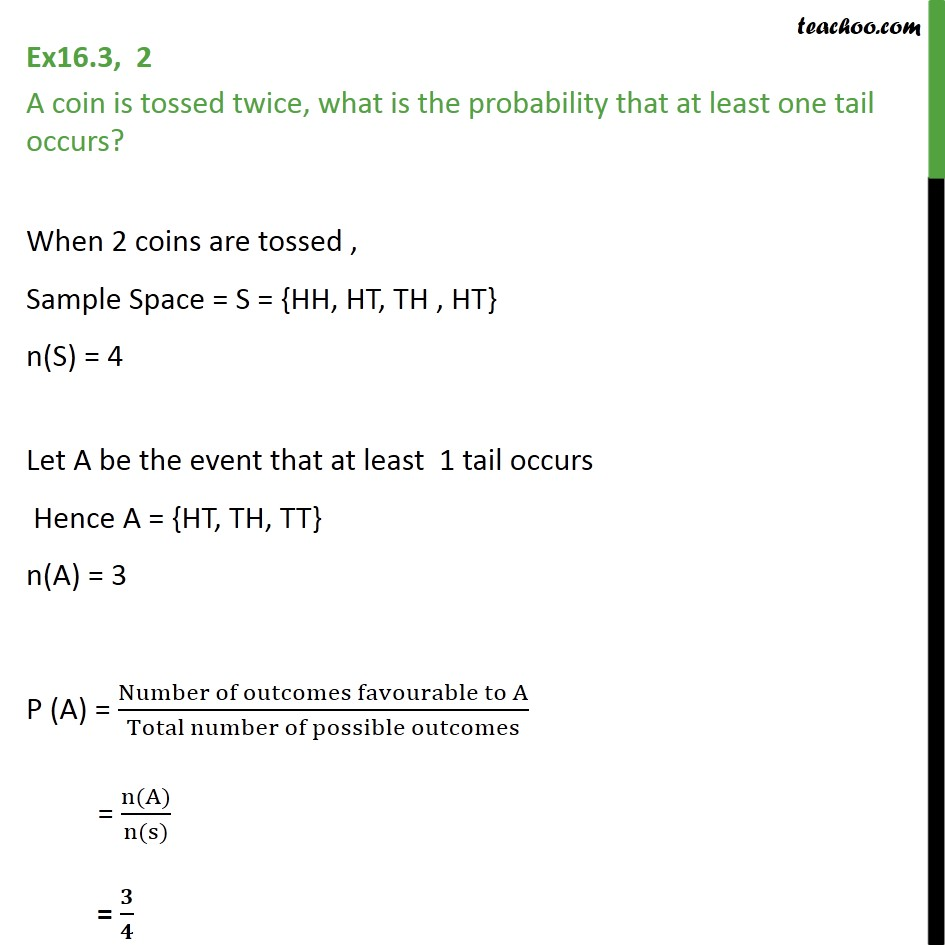Ex 14.2

Chapter 14 Class 11 Probability
Serial order wiseLearn in your speed, with individual attention - Teachoo Maths 1-on-1 Class

### Transcript

Ex 14.2, 2 A coin is tossed twice, what is the probability that at least one tail occurs? When 2 coins are tossed , Sample Space = S = {HH, HT, TH , HT} n(S) = 4 Let A be the event that at least 1 tail occurs Hence A = {HT, TH, TT} n(A) = 3 P (A) = Number of outcomes favourable to A﷮Total number of possible outcomes﷯ = n(A)﷮n(s)﷯ = 𝟑﷮𝟒﷯